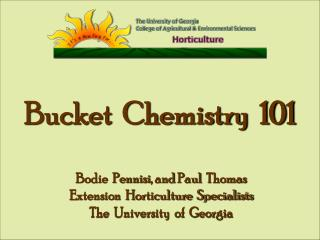DownloadDownload PresentationBucket Chemistry 101

# Bucket Chemistry 101

Download Presentation## Bucket Chemistry 101

- - - - - - - - - - - - - - - - - - - - - - - - - - - E N D - - - - - - - - - - - - - - - - - - - - - - - - - - -
##### Presentation Transcript

1. Bucket Chemistry 101 Bodie Pennisi, and Paul Thomas Extension Horticulture Specialists The University of Georgia

2. Bucket Chemistry

3. Fertilizer Problems Are Not That Bad !

4. The Way To Tackle Them Is …

5. Greenhouse Crops and Fertilizer Recommendations

6. Fertilizer Stock Tank

7. PROPORTIONER A device used in a fertigation system to control the rate of fertilizer applied through the system.

8. Fertilizer Injector or Proportioner Stock Fertilizer Tank 1 part stock solution Water line 100 parts of water Injector ratio 1 : 100 Irrigation line to plants

9. Fertilizer Injector or Proportioner Stock Tank Water line 1 part stock solution 5 gal 25 50 100 200 500 etc. x parts of water Injector ratios 2% 1% 0.5% 0.1% 1:50 = 1:100 = 1:200 = 1:500 = To get from ratios to percent: (1/50) x 100 = 2% To get from percent to ratios : 100/2%=1:50

10. Fertilizer recommendations for greenhouse crops are given as either ppm of a specific fertilizer nutrient or in pounds and ounces (weight basis) of a fertilizer formulation per 100 gallons of water Parts per million (ppm) One part of a substance into one million parts of another substance

11. Things You Need To Know To Solve ppm Problems: • Recommended fertilizer application rate. • Example: 8 ounces per 100 gal or 200 ppm nitrogen • 2. Fertilizer formulation and analysis. • Example: 20-10-20 • Injection ratio • Example: 1:100 • Size of stock tank in gallons • Example: 25 gallons

12. Weight Basis Example: A chrysanthemum grower gets a recommendation to apply 20-20-20 water-soluble formulation to a final concentration of 16 oz per 100 gallons of water. How much fertilizer should be mixed in a 25 gallon stock tank if an injector with a 1:30 injection ratio will be used?

13. Things You Need To Know To Solve This Problem: • Recommended fertilizer application rate. • 16 ounces per 100 gal • 2. Fertilizer formulation and analysis. • 20-20-20 • Injection ratio • 1:30 • Size of stock tank in gallons • 25 gallons

14. Solution Step 1: Adjust the rate for the stock tank size using the following equation: Equation 1 oz per 100 gal = oz fertilizer per stock tank 100 / Stock Tank Size (gal) 16.0 oz per 100 gal= 4.0 oz of 20-20-20 100 / 25 gal

15. Solution Step 2: Adjust the rate for the injection ratio: Equation 2 oz per gallon x injector ratio = oz per stock tank 4.0 oz per 25 gal x 30 = 120.0 oz per 25 gal tank!

16. Commercial Fertilizer (ppm) Example: A pansy grower gets a recommendation to apply 150 ppm nitrogen using 20-10-20 water-soluble formulation. How much fertilizer should be mixed in a 25 gallon stock tank if an injector with a 1:100 injection ratio will be used?

17. Things You Need To Know To Solve This Problem: • Recommended fertilizer application rate. • 150 ppm nitrogen • 2. Fertilizer formulation and analysis. • 20-10-20 • Injection ratio • 1:100 • Size of stock tank in gallons • 25 gallons

18. The Rule of 75 1 oz / 100 gal = 75 ppm • HOW DID WE GET THIS ? One ounce (28 grams) of any pure dry substance that will dissolve 100% in a volume of 100 gal equals 75 ppm 1 oz = 28 g or 28,000 mg 28,000 mg ÷ 379 L = 73.88 ppm ( 1 gal = 3.79 L 100 gal = 379 L )

19. Solution Step 1: Convert the ppm recommendation to a weight basis using the following equation: Equation 3 Recommended ppm x dilution factor = oz fertilizer/gal stock the N number (% ) of fertilizer X 75 150 ppm x 100 = 10.0 oz of 20-10-20 per gal stock 20 x 75 (final solution)

20. Solution Step 2: Use equation 1 to adjust for a stock tank size of 25 gallons: (oz per gal stock x (# gallons in stock tank) = Oz fertilizer per stock tank 10.0 oz x 25 gal tank = 250 oz of 20-10-20 in the tank

21. Solution Each bag is 25 lbs! Remember 25 lbs x 16 ox…the number of bags needed = 250 oz/ (16 oz X 25lbs ) or 0.62 bags! Step 4: Convert ounces to pounds and ounces where: 16 ounces = 1 pound (dry) 250.0 oz per 25 gal = 15 lbs 10 oz 20-10-20 16 oz/lb (. . . .in a 25 gallon stock tank) This will give you 150 ppm with a 1/100 ratio